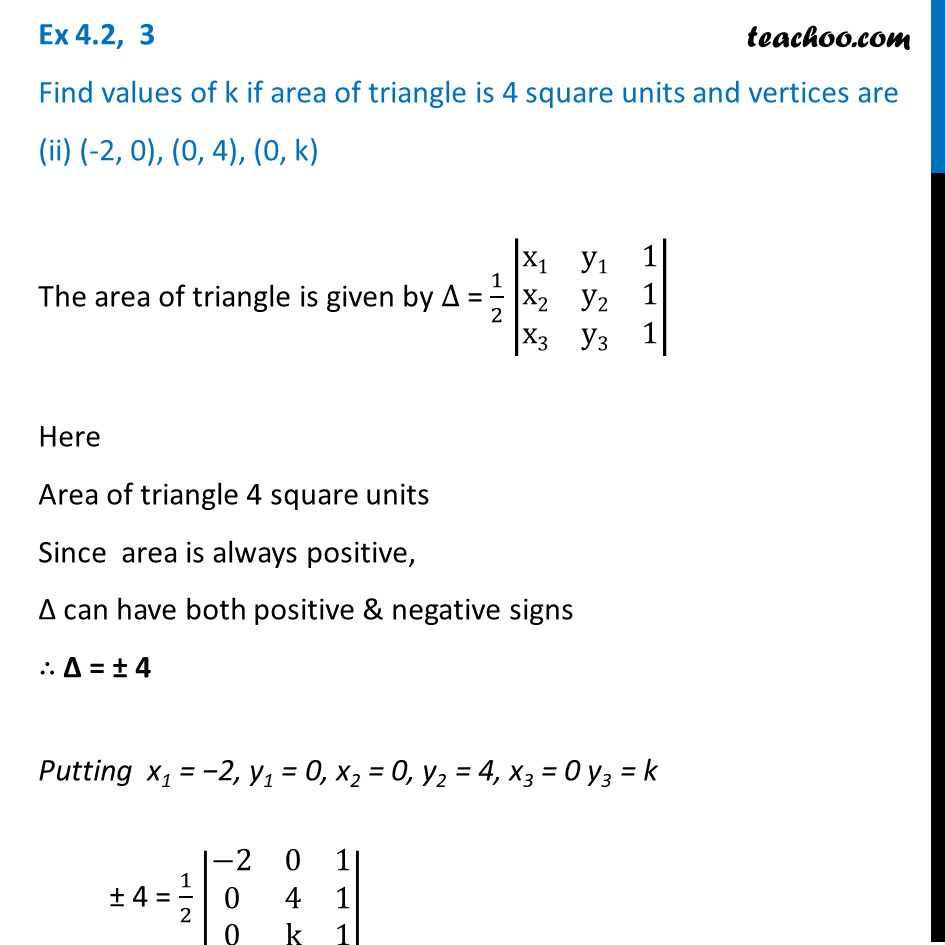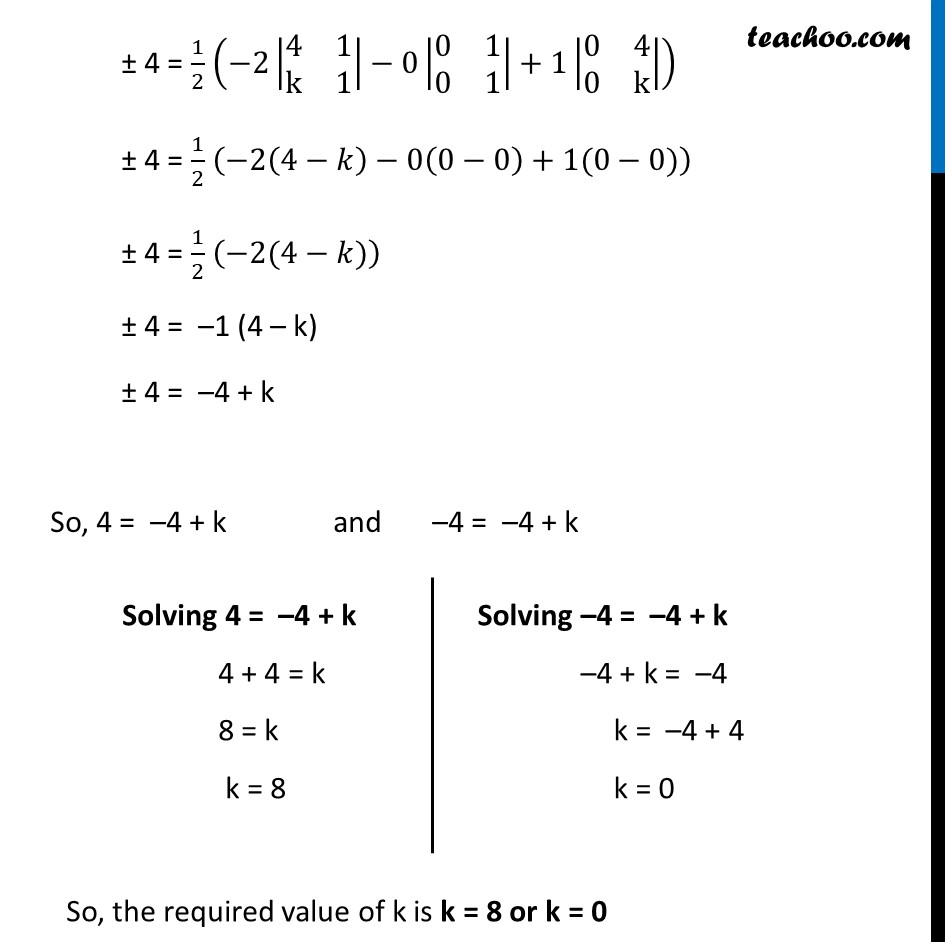Ex 4.2

Chapter 4 Class 12 Determinants
Serial order wiseLearn in your speed, with individual attention - Teachoo Maths 1-on-1 Class

### Transcript

Ex 4.2, 3 Find values of k if area of triangle is 4 square units and vertices are (ii) (-2, 0), (0, 4), (0, k) The area of triangle is given by ∆ = 1/2 |■8(x1&y1&1@x2&y2&1@x3&y3&1)| Here Area of triangle 4 square units Since area is always positive, ∆ can have both positive & negative signs ∴ Δ = ± 4 Putting x1 = −2, y1 = 0, x2 = 0, y2 = 4, x3 = 0 y3 = k ± 4 = 1/2 |■8(−2&0&1@0&4&1@0&k&1)| ± 4 = 1/2 (−2|■8(4&1@k&1)|−0|■8(0&1@0&1)|+1|■8(0&4@0&k)|) ± 4 = 1/2 (−2(4−𝑘)−0(0−0)+1(0−0)) ± 4 = 1/2 (−2(4−𝑘)) ± 4 = –1 (4 – k) ± 4 = –4 + k So, 4 = –4 + k and –4 = –4 + k Solving 4 = –4 + k 4 + 4 = k 8 = k k = 8 Solving –4 = –4 + k –4 + k = –4 k = –4 + 4 k = 0 So, the required value of k is k = 8 or k = 0 Ex 4.2, 4 (i) Find equation of line joining (1, 2) and (3, 6) using determinants Let L be the line joining the points (1, 2) & (3, 6) Let (x, y) be the third point on line Since all the there point lie on the same line, they do not from a triangle Hence, Area of triangle = 0 Thus, ∆ = 0 3 points collinear Area of triangle = 0 Area of triangle ≠ 0 We know that Area of triangle is given by ∆ = 1/2 |■8(x1&y1&1@x2&y2&1@x3&y3&1)| Here, x1 = x , y1 = y x2 = 1 , y2 = 2 x3 = 3 , y3 = 6 & ∆ = 0 Putting values 0 = 1/2 |■8(𝑥&𝑦&1@1&2&1@3&6&1)| 0 = 1/2 (x|■8(2&1@6&1)|−y|■8(1&1@3&1)|+1|■8(1&2@3&6)|) 0 = 1/2 (x (2 – 6) – y (1 – 3) + 1 (6 – 6)) 0 = 1/2 (x (–4) – y (–2) + 1(0)) 0 = 1/2 ( –4x + 2y + 0 ) 0 = –2x + y 2x – y = 0 y = 2x Thus, the required equation of line joining is y = 2x